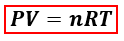# Problem: The following equation shows the combustion of methane.CH4 (g) + 2O2(g) → CO2(g) + 2H2O(g)If 6.0 g of CH4 reacts completely, what is the final pressure of the CO 2 produced and collected in a 1.4 L container at a temperature of 21°C?

###### FREE Expert Solution
95% (468 ratings)
###### FREE Expert Solution

We are asked to calculate the final pressure of CO2 produced from the combustion of CH4. We can calculate the pressure of the gas using the ideal gas equation:P = pressure, atm
V = volume, L
n = moles, mol
R = gas constant = 0.08206 (L·atm)/(mol·K)
T = temperature, K

In the ideal gas equation, the amount of CO2 in moles is needed but the mass of the CH4 is given instead. We will have to calculate the moles of CO2 produced from CH4 first using the balanced reaction equation and mole to mole comparison.

95% (468 ratings)###### Problem Details

The following equation shows the combustion of methane.

CH4 (g) + 2O2(g) → CO2(g) + 2H2O(g)

If 6.0 g of CH4 reacts completely, what is the final pressure of the CO 2 produced and collected in a 1.4 L container at a temperature of 21°C?

Frequently Asked Questions

What scientific concept do you need to know in order to solve this problem?

Our tutors have indicated that to solve this problem you will need to apply the Gas Stoichiometry concept. You can view video lessons to learn Gas Stoichiometry. Or if you need more Gas Stoichiometry practice, you can also practice Gas Stoichiometry practice problems.

What is the difficulty of this problem?

Our tutors rated the difficulty ofThe following equation shows the combustion of methane.CH4 (...as medium difficulty.

What professor is this problem relevant for?

Based on our data, we think this problem is relevant for Professor Gellene's class at TTU.